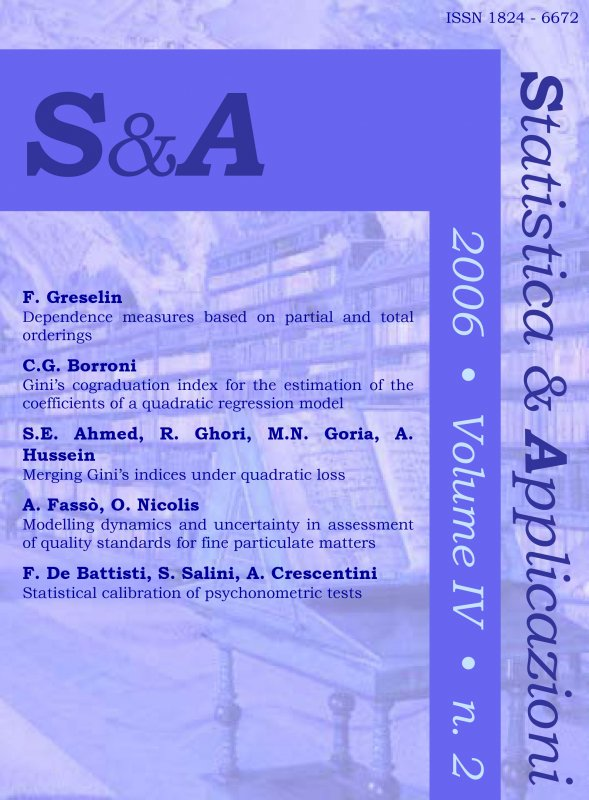Your browser does not support JavaScript!

Gini’s cograduation index for the estimation of the coefficients of a quadratic regression model

digitalArticle
 journal STATISTICA & APPLICAZIONI issue STATISTICA & APPLICAZIONI - 2006 - 2 title Gini’s cograduation index for the estimation of the coefficients of a quadratic regression model author Claudio Giovanni Borroni publisher Vita e Pensiero formatArticle |Pdf language English online since 06-2016 issn 18246672 (print) Write a comment for this product

Ebook format Pdf readable on these devices:

• Computer
with Software:
• Android
with App:
• Ibs Tolino
• Kobo
Least square estimates of regression parameters may become unreliable when some outliers affect the data. This fact forces to search for different methods of estimation, some of which consist of substituting ranks to observations to avoid influences by extremes values. Cifarelli (1978) proposes one of such methods to estimate the slope parameter of a linear regression model, by using Gini’s cograduation index. In this paper Cifarelli’s method is generalized to the case of quadratic regression, that is to the estimation of the parameters β and γ of the model Yi = α + β xi + γ xi2 + εi (i = 1 ,…, n) where the xi’s are supposed to be known constants and the εi’s are iid error terms following an unknown distribution. The parameters β and γ are estimated in a two-step procedure, each time by searching for the value which sets to zero the Gini’s cograduation index between the residuals of the model and the values of the explanatory variable (in analogy with a related property of the least square method involving covariance). A simulation study is provided to test the performance of the proposed method, which proves to be often superior to other known related methodologies.

Author biography

Claudio Giovanni Borrone, Dipartimento di Metodi Quantitativi per le Scienze Economiche ed Aziendali – Università degli Studi di Milano Bicocca – pza Ateneo Nuovo, 1 20126 MILANO (e-mail: claudio.borroni@unimib.it).